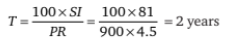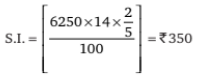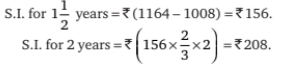# Mathematical Aptitude - Interest

>>>>>>>>Interest

• A

2 years• B

3 years• C

1 year• D

4 years• Option : A
• Explanation : P = Rs 900
S.I. = Rs 81
T = ?
R = 4.5%• A

Rs 350• B

Rs 450• C

Rs 550• D

Rs 650• Option : A
• Explanation : P = Rs 6250, R = 14 % and T = (146/365) years = 2/5 years.• A

800,14%• B

800,13%• C

800,12%• D

800,19%• Option : B
• Explanation :Therefore, principal = Rs (1008 – 208) = Rs 800.
Now, P = 800, T= 2 and S.I. = 208.
Therefore, rate = (100 × S.I.)/(P × T) = [(100 × 208)/(800 × 2)]% = 13%

• A

Rs 600• B

Rs 666• C

Rs 780• D

Rs 800• Option : D
• Explanation : Let the sum be Rs a.
Then we can write: [{x × 10 × 7}/{100 × 2}] – [{x × 12 × 5}/{100 × 2}] = 40. This can be written as:
7x/20 – 3x/10 = 40.
Therefore, we have x = Rs 800.

• A

Rs 12,000• B

Rs 11,000• C

Rs 14,000• D

Rs 15,000• Option : D
• Explanation : Here, we have, the principal = Rs [{100 × 5400}/{12 × 3}] = Rs 15000.
Thus, the correct option is (D).

## Description

• Simple Interest Questions can be used to give quizzes by any candidate who is preparing for UGC NET Paper 1
• This Simple Interest Questions section will help you test your analytical skills in a tricky method, thereby giving you an edge over other students
• All candidates who have to appear for the Kendriya Vidyalaya Entrance exam can also refer to this mcq section.
• You can also get access to the Simple Interest MCQ ebook.
• Simple Interest Questions can be used in the preparation of JRF, CSIR, and various other exams.
• You can also download pdf for these Simple Interest multiple-choice questions Answers.
• This Simple Interest Multiple Choice Questions Answers section can also be used for the preparation of various competitive exams like UGC NET, GATE, PSU, IES, and many more.
• Simple Interest Questions can be used to gain a credit score in various undergraduate and postgraduate courses like Bcom, Mcom and more

Related Quiz.
Interest